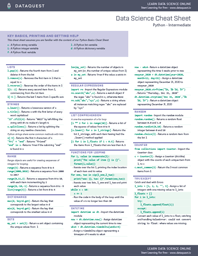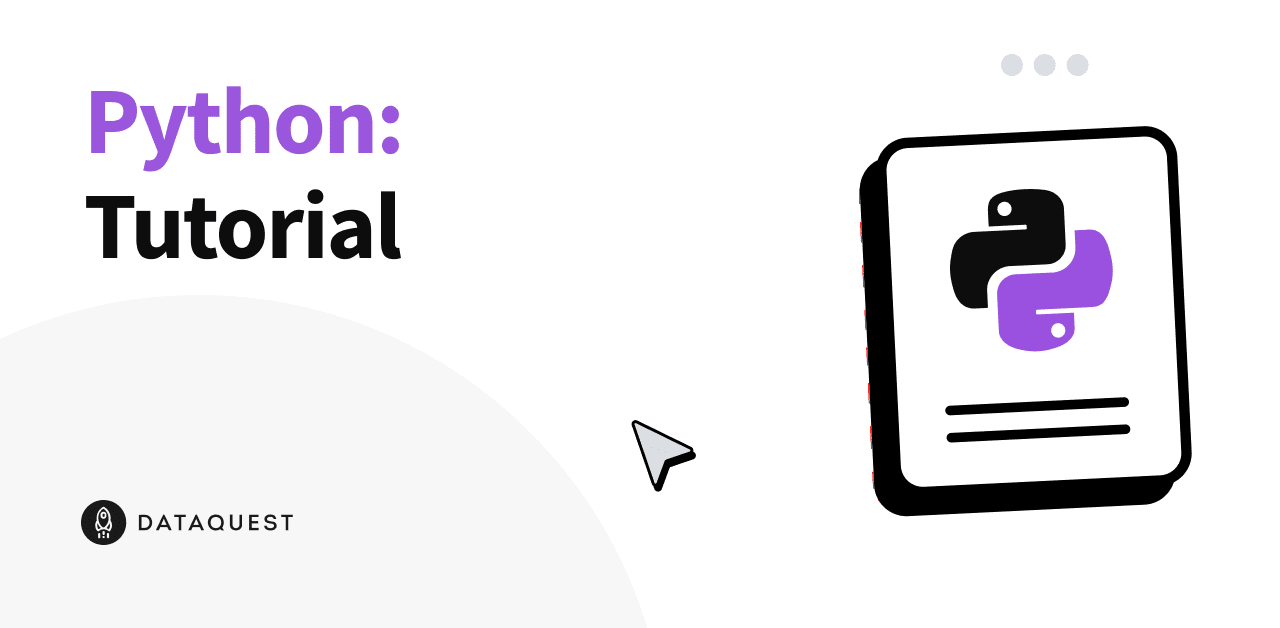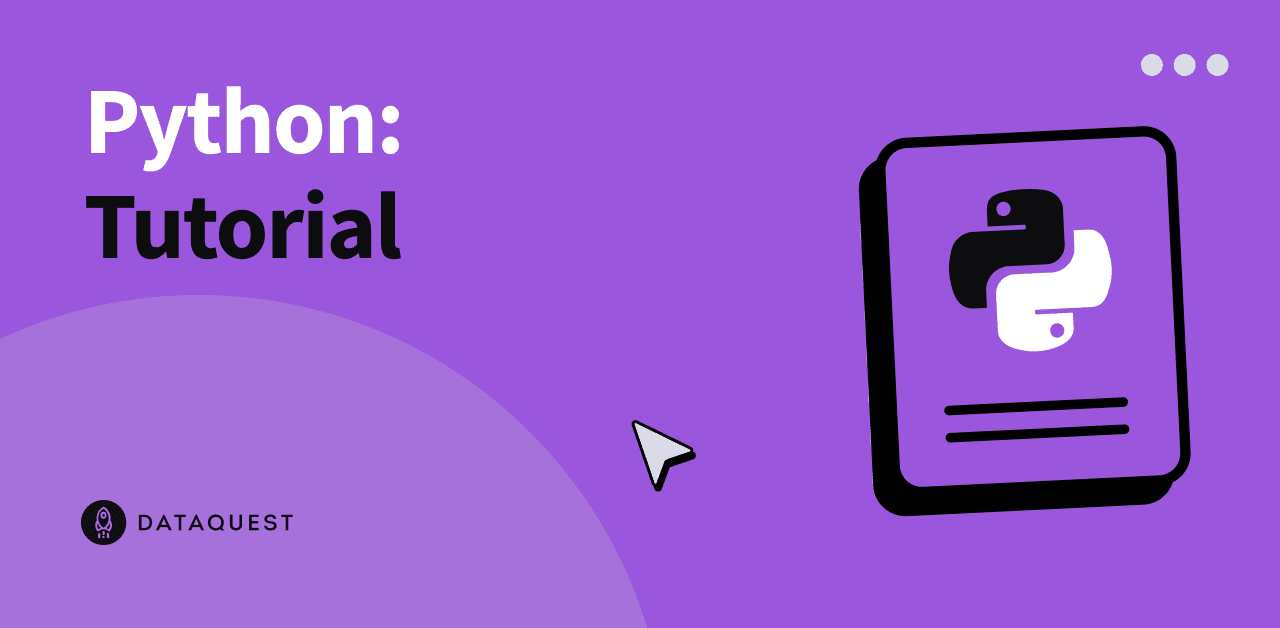August 29, 2017

# Python Cheat Sheet for Data Science: IntermediateThe printable version of this cheat sheet

The tough thing about learning data is remembering all the syntax. While at Dataquest we advocate getting used to consulting the Python documentation, sometimes it's nice to have a handy reference, so we've put together this cheat sheet to help you out!

This cheat sheet is the companion to our Python Basics Data Science Cheat Sheet

If you'd like to learn Python, we have a Python Programming: Beginner course which can start you on your data science journey.

## Key Basics, Printing and Getting Help

This cheat sheet assumes you are familiar with the content of our Python Basics Cheat Sheet.

`s` | A Python string variable `i` | A Python integer variable `f` | A Python float variable `l` | A Python list variable `d` | A Python dictionary variable

## Lists

`l.pop(3)` | Returns the fourth item from `l` and deletes it from the list `l.remove(x)` | Removes the first item in `l` that is equal to `x` `l.reverse()` | Reverses the order of the items in `l` `l[1::2]` | Returns every second item from `l`, commencing from the 1st item `l[-5:]` | Returns the last `5` items from `l`

## Strings

`s.lower()` | Returns a lowercase version of `s` `s.title()` | Returns `s` with the first letter of every word capitalized `"23".zfill(4)` | Returns `"0023"` by left-filling the string with `0`'s to make it's length `4`. `s.splitlines()` | Returns a list by splitting the string on any newline characters. Python strings share some common methods with lists| `s[:5]` | Returns the first `5` characters of `s` `"fri" + "end"` | Returns `"friend"` `"end" in s` | Returns `True` if the substring `"end"` is found in `s`

## Range

Range objects are useful for creating sequences of integers for looping.

`range(5)` | Returns a sequence from `0` to `4` `range(2000,2018)` | Returns a sequence from `2000` to `2017` `range(0,11,2)` | Returns a sequence from `0` to `10`, with each item incrementing by `2` `range(0,-10,-1)` | Returns a sequence from `0` to `-9` `list(range(5))` | Returns a list from `0` to `4`

## Dictionaries

`max(d, key=d.get)` | Return the key that corresponds to the largest value in `d` `min(d, key=d.get)` | Return the key that corresponds to the smallest value in `d`

## Sets

`my_set = set(l)` | Returns a set object containing the unique values from `l` `len(my_set)` | Returns the number of objects in `my_set` (or, the number of unique values from `l`) `a in my_set` | Returns `True` if the value `a` exists in `my_set`

## Regular expressions

`import re` | Import the Regular Expressions module `re.search("abc",s)` | Returns a `match` object if the regex `"abc"` is found in `s`, otherwise `None` `re.sub("abc","xyz",s)` | Returns a string where all instances matching regex `"abc"` are replaced by `"xyz"`

## List comprehension

A one-line expression of a for loop

`[i ** 2 for i in range(10)]` | Returns a list of the squares of values from `0` to `9` `[s.lower() for s in l_strings]` | Returns the list `l_strings`, with each item having had the `.lower()` method applied `[i for i in l_floats if i < 0.5]` | Returns the items from `l_floats` that are less than `0.5`

## Functions for looping

``````for i, value in enumerate(l):
print("The value of item {} is {}".format(i,value))``````

Iterates over the list l, printing the index location of each item and its value

``````for one, two in zip(l_one,l_two):
print("one: {}, two: {}".format(one,two))``````

Iterates over two lists, `l_one` and `l_two` and print each value

``````while x < 10:
x += 1``````

Runs the code in the body of the loop until the value of `x` is no longer less than `10`

## Datetime

`import datetime as dt` | Imports the `datetime` module `now = dt.datetime.now()` | Assigns `datetime` object representing the current time to `now` `wks4 = dt.datetime.timedelta(weeks=4)` | Assigns a `timedelta` object representing a timespan of 4 weeks to `wks4` `now - wks4` | Returns a `datetime` object representing the time 4 weeks prior to `now` `newyear_2020 = dt.datetime(year=2020, month=12, day=31)` | Assigns a `datetime` object representing December 25, 2020 to `newyear_2020` ```newyear_2020.strftime( dt.datetime.strptime('Dec 31, 2020', ```

## `Random`

``` import random | Imports the random module random.random() | Returns a random float between 0.0 and 1.0 random.randint(0,10) | Returns a random integer between 0 and 10 random.choice(l) | Returns a random item from the list l Counter from collections import Counter | Imports the Counter class c = Counter(l) | Assigns a Counter (dict-like) object with the counts of each unique item from l, to c c.most_common(3) | Returns the 3 most common items from l Try/Except Catch and deal with errors l_ints = [1, 2, 3, "", 5] Assigns a list of integers with one missing value to l_ints l_floats = [] for i in l_ints: try: l_floats.append(float(i)) except: l_floats.append(i) Converts each value of l_ints to a float, catching and handling ValueError: could not convert string to float: where values are missing. Download a printable version of this cheat sheet If you'd like to download a printable version of this cheat sheet you can do so below. Download a Printable PDF of this Cheat Sheet Cheat Sheets ```
``` ```
```About the author Celeste Grupman Celeste is the Director of Operations at Dataquest. She is passionate about creating affordable access to high-quality skills training for students across the globe. You May Also LikeHow Long Does It Really Take to Learn Python? (2023 Version) READ MORETutorial: Demystifying Python Lists READ MORE ```
``` Learn data skills for freeJoin 1M+ learners Try free courses PythonRSQLPower BI ```
``` ```
``` ```
``` ```
```All rights reserved 2023 - Dataquest Labs, Inc. Terms of Use Privacy Policy About For Business For Educators About Dataquest Contact Us Success Stories Partnership Programs Sitemap Become Data Scientist in Python Data Analyst in R Data Engineer Data Analyst in Python Learn Course Catalog Learn Python Learn R Learn Data Science Learn Power BI Learn SQL Learn Data Analysis Courses Machine Learning Courses SQL Courses Data Analysis Courses Python Courses Business Analysis Courses Data Visualization Courses Data Engineering Courses Data Science Courses Sign In Start Free Dashboard Learning Path Catalog Full Catalog Career Paths Skill Paths Individual Courses Understanding Data Roles Resources A Better Way to Learn Success Stories Blog Live Tutorials For Teams ```